## Introduction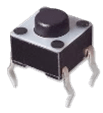The using of a push button will help you to understand how the input works in Arduino Uno.

Be aware, even if the push button has 4 pins, it’s a dipole. In fact, the pins are linked two by two.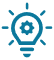### Advice to not plugged in the wrong way

The pins in diagonals of the push button are not always connected together. Therefore, to not make mistake of sense you can choose the two diagonals. (We use that in our circuits).You can choose the color of each button thanks to this kit.

Also, you can change the size like this one.

## Pick up the state of the push button

### 1) By using the resistor pull-up external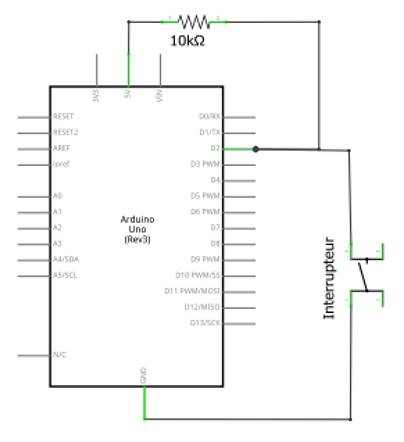To connect the push button, we link the supply (5V) and the ground (GND). To pick up the state of the push button, we link to the pins 2. You can’t link directly the supply to the Arduino card, we need a resistor.

If the push button is pushed, the serial monitor display 0. Otherwise, it displays 1.
``````const int pin_push_Button = 2; // Push button attached to pin 2

void setup() {
Serial.begin(9600); // Initialized with the serial monitor
}

void loop() {
delay(1000) ; // wait 1 second
boolean State_button = digitalRead(pin_push_Button) ; // Pick up the state of the push button
Serial.println(State_button) ; // display the state of the serail monitor
}``````

### 2) By using the resistor pull-up internal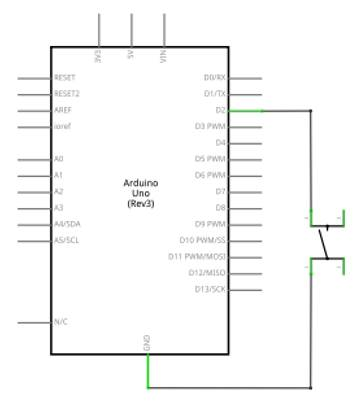To simplify the circuits, it is possible to modify the program for that the pull-up is inside the Arduino Card.

``````const int pin_push_Button = 2; // Push button attached to pin 2

void setup() {
Serial.begin(9600); // Initialized with the serial monitor
pinMode(pin_push_Button, INPUT_PULLUP); // Initialized the push button in input pull-up
}

void loop() {
delay(1000) ; // wait 1 second
boolean State_button = digitalRead(pin_push_Button) ; // Pick up the state of the push button
Serial.println(State_button) ; // display the state of the serail monitor
}``````
pinMode(2, INPUT_PULLUP) permits you to initialize the pin 2 as an input with the resistor of pull up.

## Turn on the LED with a push buttonWhen we push the button, the led turn on. When we release it, the led turn off.
``````const int pin_LED = 2; // The LED is atatched to the pin 2

void setup() {
pinMode(pin_LED, OUTPUT); // We initialized the led
}
void loop(){
}``````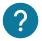### Why is it so important to put a resistor ?

The supply of the Arduino is 5V, therefore it’s required to put a resistor to not burnt the led. One advice is using a resistor of 220 ohms.### Why pinMode(2, OUTPUT) ?

We initialize the led on the pin 2 of the Arduino card. We choose output because the signal go out of the Arduino card to control the LED.

With this circuit you have to push the button to see the LED turn on. This is not really practical, therefore you have to use a switch. In fact, the switch will keep the state unlike the push button. That’s mean you don’t to stay with your finger pressed on the push button to see the light turn on with a switch.

## Transform a push button into a switch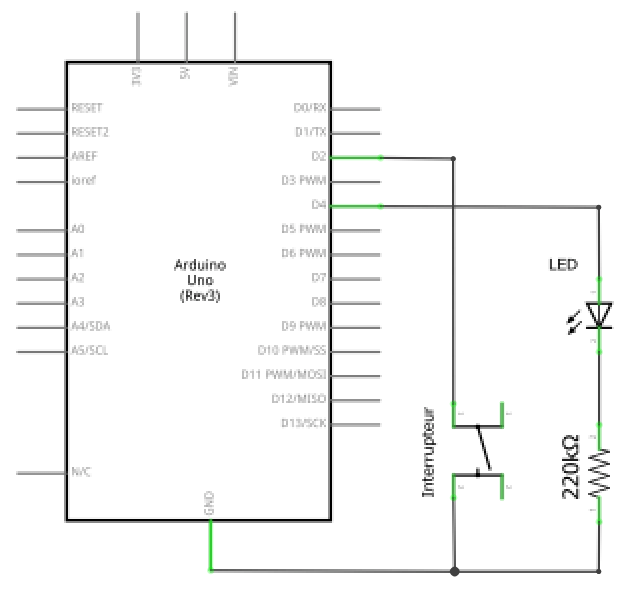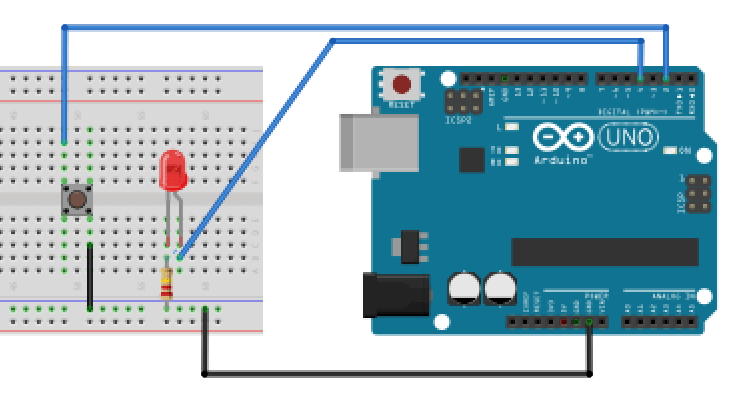``````const int pin_switch = 2; // The pin of the push button
const int pin_LED = 4; // The pin of the led
boolean led_turnon = 0; // A boolean to turn the led on and off
boolean push_button = 0; // A boolean to store the state

void setup() {
pinMode(pin_switch, INPUT_PULLUP); // The pin of the switch initialized to a pullup
pinMode(pin_LED, OUTPUT); // The led is initialized as an output
Serial.begin(9600); //We initialized the serial monitor
}

voidloop() {
boolean state_button = digitalRead(pin_switch) ; // Boolean to store the state of the push button
if(state_button == 0 and push_button == 0) { // When you push the button
push_button = 1;
if(led_turnon == 0) { // If the led is turn off we turn on it
digitalWrite(pin_LED, HIGH);
Serial.println(“turn on”) ;
led_turnon = 1;
}
else { // If the led is turn on we turn off it.
digitalWrite(pin_LED, LOW);
led_turnon = 0;
Serial.println(“turn off“);
}
}
if(state_button == 1 and push_button == 1) { //When you stop to press the push button
push_button = 0;
}
delay(10) ; // Wait 10 ms
}``````

## Transform a push button into a switch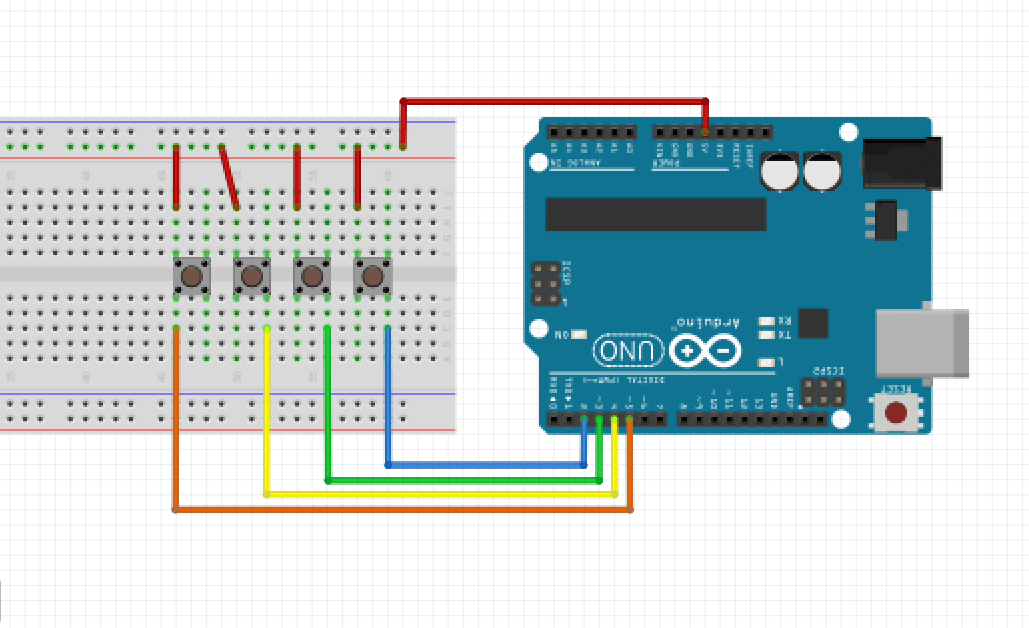A push button has unexpected functionalities. This is an example of it : We will use the push buttons as keypad.
To know if the password taped by the user is good you have to check the serial monitor.

``````int code[] = {1,1,1,1}; // The code (the code has to be composed of 0 and 1)

int tab_pin[]={2,3,4,5}; // table to store the pins of the 4 push buttons
int tab_val[]={0,0,0,0}; //table to read the state of the 4 push buttons
int tab_val_stock[]={0,0,0,0}; //table to read the state of the 4 push buttons
int changement = 0; // = 0 if no push buton has change state
int bouton = -1;
int a = 0; //variable to verify that the code is good
int b = 0; // variable to write "code succed" many times

void setup() {
Serial.begin(9600);
for (int i=0 ; i<4 ; i++) { // loop to initialize the pins of the 4 push buttons
pinMode(tab_pin[i], OUTPUT);
}
}

void loop() {
for(int i=0 ; i<4 ; i++) {
tab_val[i] = digitalRead(tab_pin[i]); // to store the state of the push button
if(tab_val[i] == 1 and bouton != i){
changement = 1;
bouton = i;
if(tab_val_stock[i] == 0){
tab_val_stock[i] = 1;
}
else {
tab_val_stock[i] = 0;
}
}
} // end for
if(tab_val[bouton] == 0){
bouton = -1;
}
// Display the state of all the push buttons if at least one change state
if(changement == 1){
Serial.println(“==”);
b = 0;
for(int i=0 ; i<4 ; i++) {
Serial.println(tab_val_stock[i]) ;
}
changement = 0;
}

// To verify the code ———————————————–
for(int i=0 ; i<4 ; i++){
if(tab_val_stock[i] != code[i]){
a = 1;
}
}
if(a == 0 and b == 0){
Serial.println(“code réussi”) ;
b = 1;
}
a = 0;

}``````

## To go further...

To simplify the circuit, you can buy a matrix module at 16 buttons

If you liked the keypad and you want to improve the system you can buy a matrix keyboard.

If you prefer a keypad with a professional look, you can buy this one.